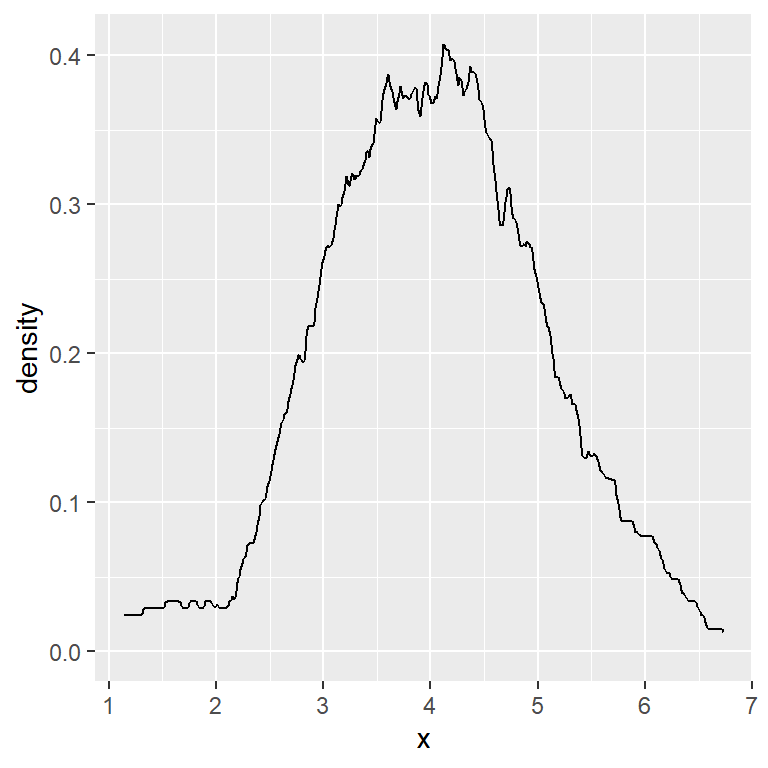# Density plot in ggplot2 with geom_density

## Density plot in ggplot2 with `geom_density`

Given a continuous variable you can create a density plot in ggplot2 with `geom_density`.

``````# install.packages("ggplot2")
library(ggplot2)

# Data
set.seed(14012021)
x <- rnorm(200, mean = 4)
df <- data.frame(x)

# Basic density plot in ggplot2
ggplot(df, aes(x = x)) +
geom_density()``````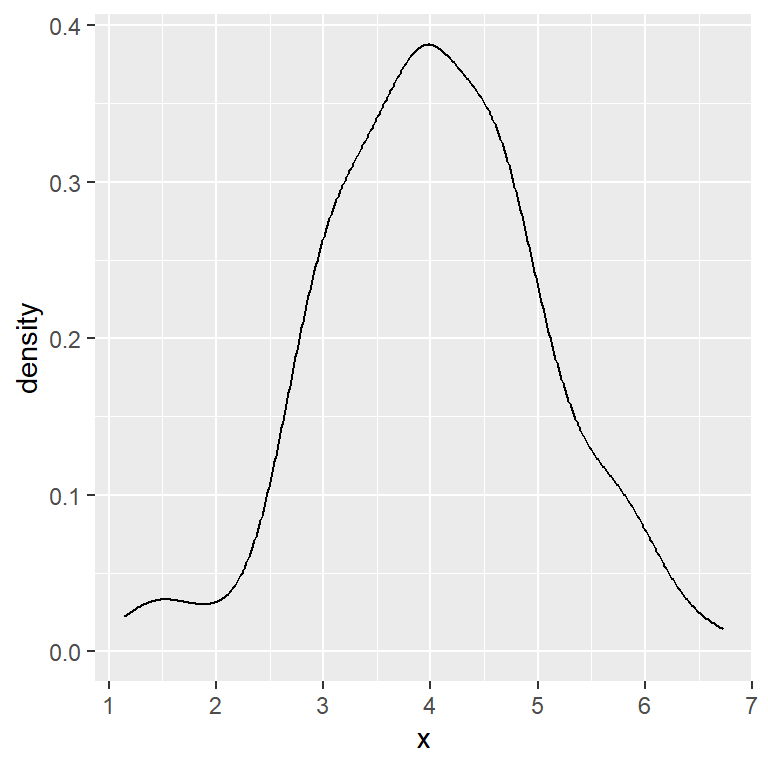The curve can be customized in several ways, such as changing its color, width or type.

``````# install.packages("ggplot2")
library(ggplot2)

# Data
set.seed(14012021)
x <- rnorm(200, mean = 4)
df <- data.frame(x)

# Density plot in ggplot2
ggplot(df, aes(x = x)) +
geom_density(color = 4,
lwd = 1,
linetype = 1)``````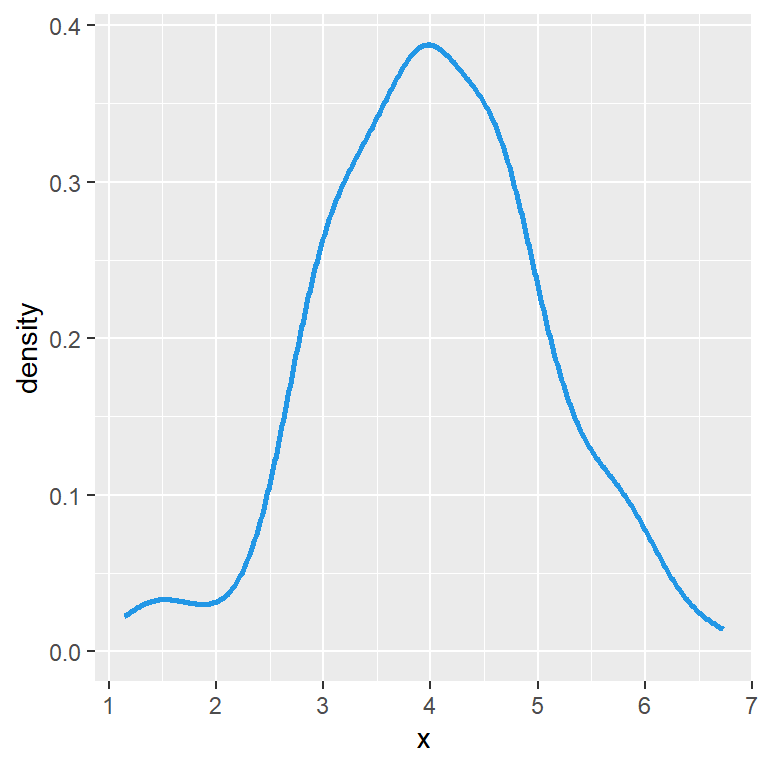You can also fill the area and change its transparency with `fill` and `alpha`, respectively.

``````# install.packages("ggplot2")
library(ggplot2)

# Data
set.seed(14012021)
x <- rnorm(200, mean = 4)
df <- data.frame(x)

# Density plot in ggplot2
ggplot(df, aes(x = x)) +
geom_density(color = 4,
fill = 4,
alpha = 0.25)``````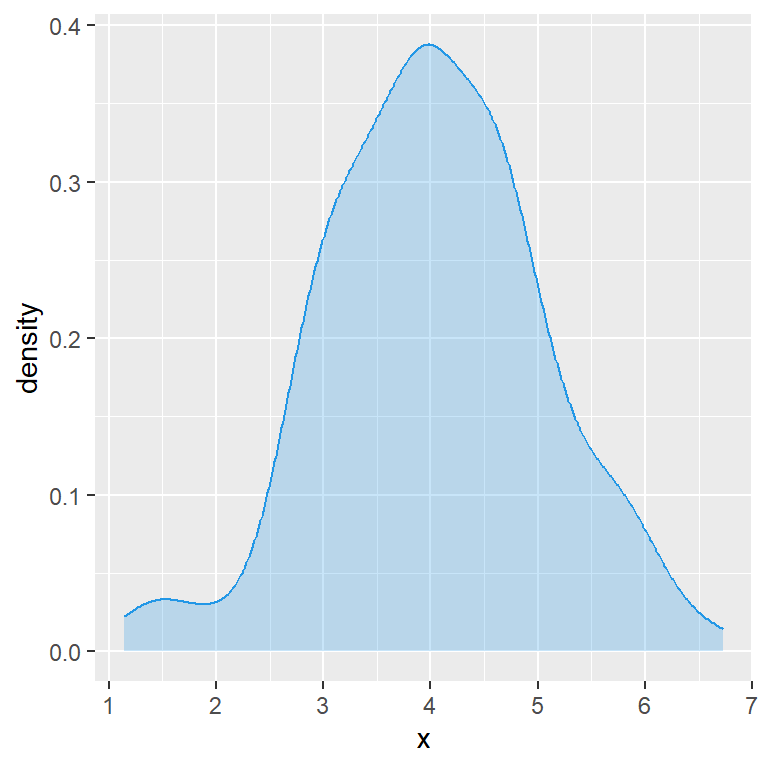## Smoothing parameter selection

When calculating a kernel density estimate a smoothing parameter (also known as bandwidth) must be selected. A big bandwidth will create a very smoothed curve, while a small bandwidth will create a sharpened curve.

The default method used for calculating the bandwidth is called rule-of-thumb, but you can choose between other options, use a bandwidth multiplier or the value you desire, as shown in the following examples.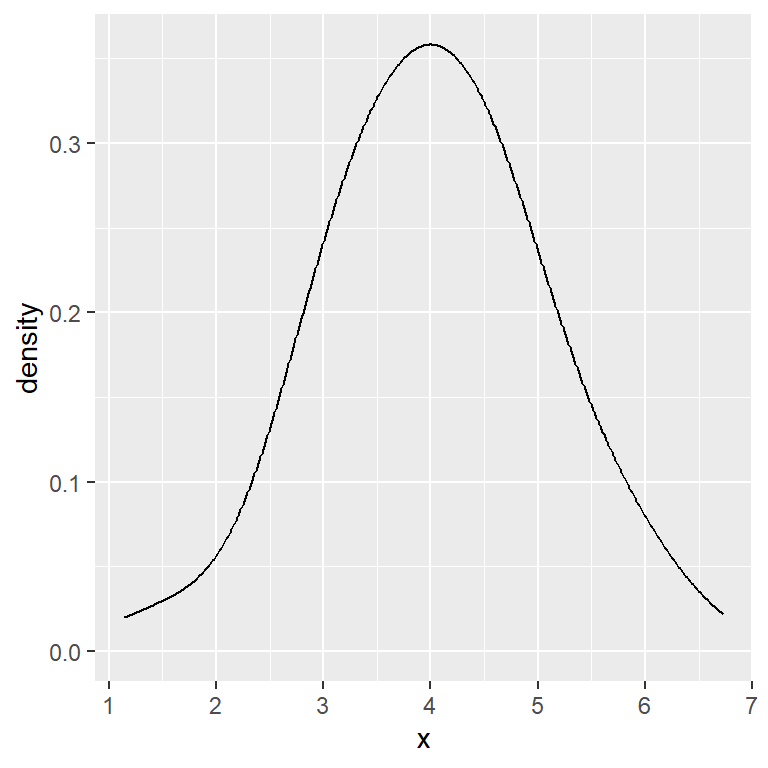Bandwidth multiplier

``````# install.packages("ggplot2")
library(ggplot2)

# Data
set.seed(14012021)
x <- rnorm(200, mean = 4)
df <- data.frame(x)

# Density plot, bandwidth multiplier
ggplot(df, aes(x = x)) +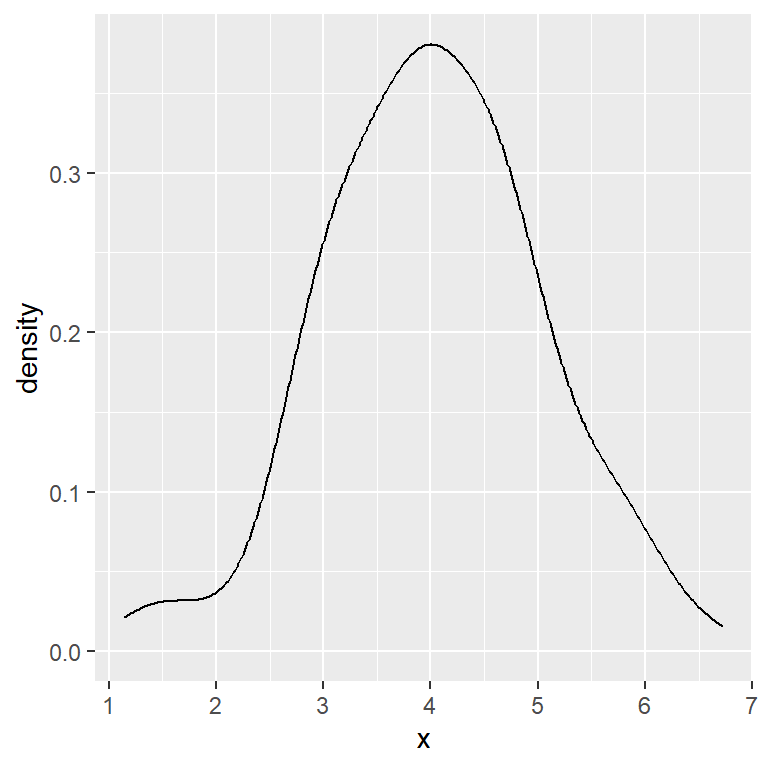Scott bandwidth (factor 1.06)

``````# install.packages("ggplot2")
library(ggplot2)

# Data
set.seed(14012021)
x <- rnorm(200, mean = 4)
df <- data.frame(x)

# Density plot, Scott bandwidth
ggplot(df, aes(x = x)) +
geom_density(bw = "nrd")``````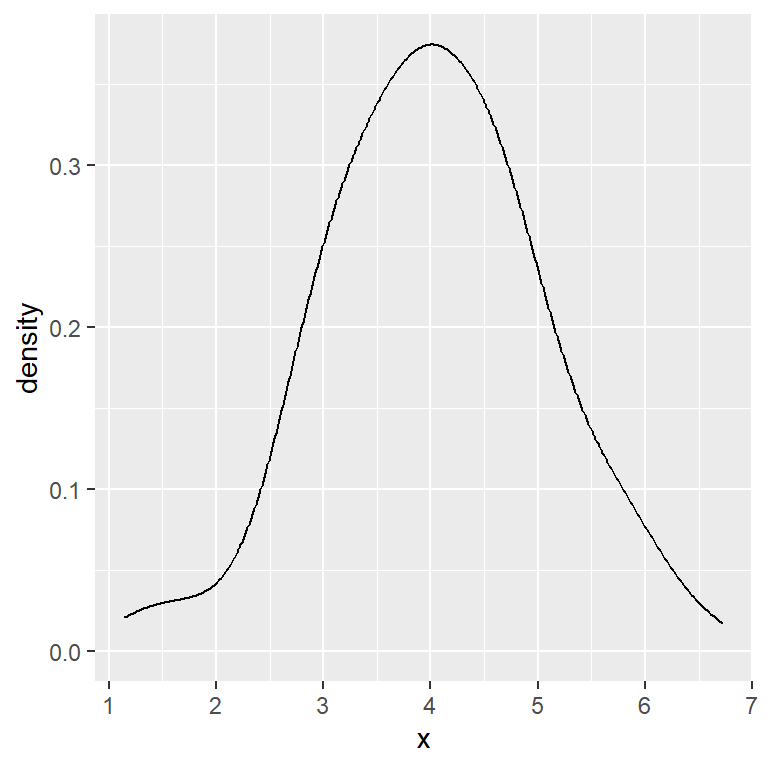Unbiased cross validation method

``````# install.packages("ggplot2")
library(ggplot2)

# Data
set.seed(14012021)
x <- rnorm(200, mean = 4)
df <- data.frame(x)

# Unbiased cross validation bandwidth
ggplot(df, aes(x = x)) +
geom_density(bw = "ucv")``````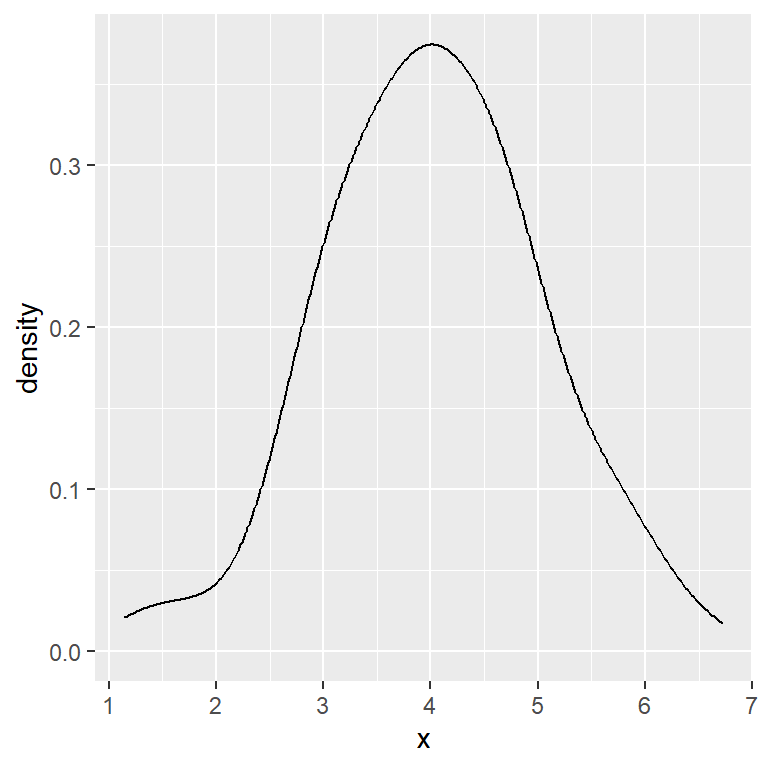Biased cross validation method

``````# install.packages("ggplot2")
library(ggplot2)

# Data
set.seed(14012021)
x <- rnorm(200, mean = 4)
df <- data.frame(x)

# Biased cross validation bandwidth
ggplot(df, aes(x = x)) +
geom_density(bw = "bcv")``````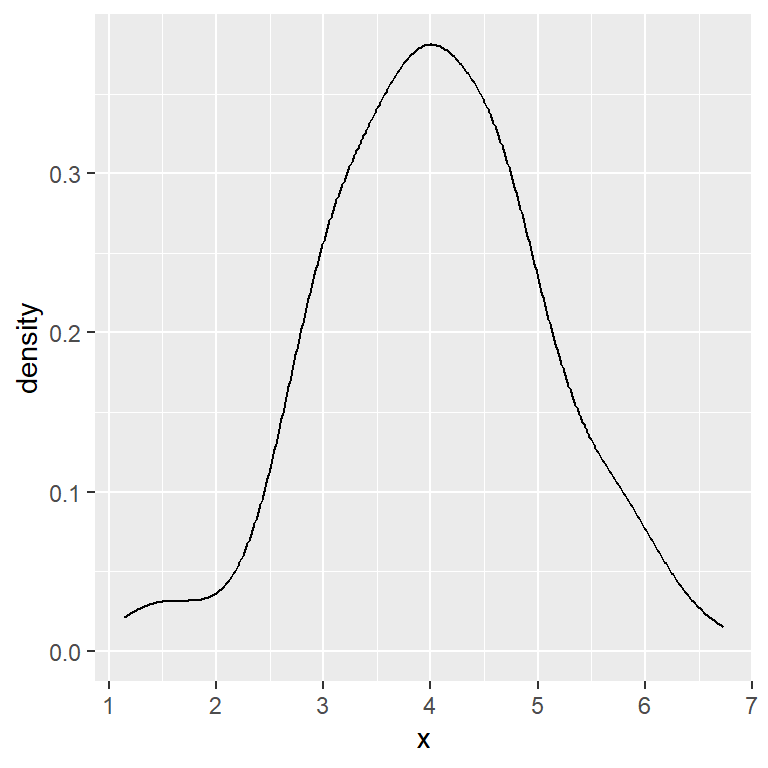Sheather & Jones method

``````# install.packages("ggplot2")
library(ggplot2)

# Data
set.seed(14012021)
x <- rnorm(200, mean = 4)
df <- data.frame(x)

# SJ bandwidth
ggplot(df, aes(x = x)) +
geom_density(bw = "SJ")``````

## Kernel selection

The kernel used can also be changed with `kernel` argument. The possible options are `"gaussian"` (default), `"rectangular"`, `"triangular"`, `"epanechnikov"`, `"biweight"`, `"cosine"` and `"optcosine"`.

Below you can see an example which uses a rectangular kernel instead of a gaussian kernel. The decision about which kernel to use will depend on your data.

Rectangular kernel

``````# install.packages("ggplot2")
library(ggplot2)

# Data
set.seed(14012021)
x <- rnorm(200, mean = 4)
df <- data.frame(x)

# Custom kernel
ggplot(df, aes(x = x)) +
geom_density(kernel = "rectangular")``````